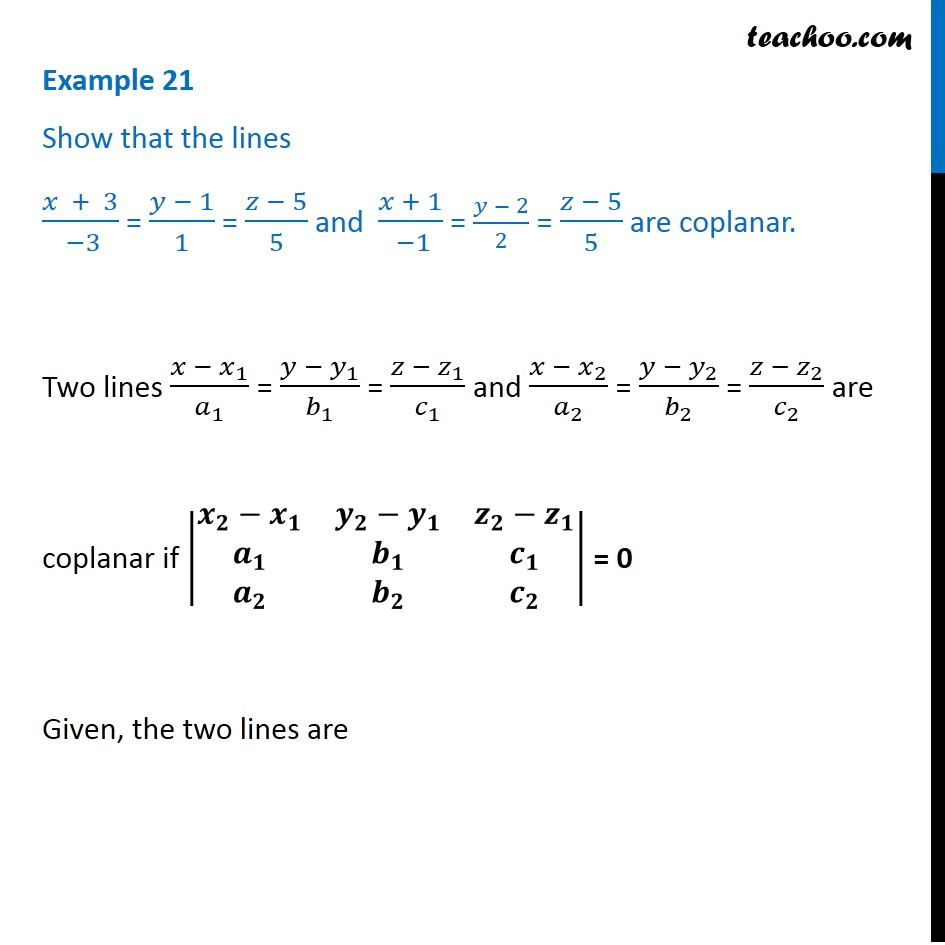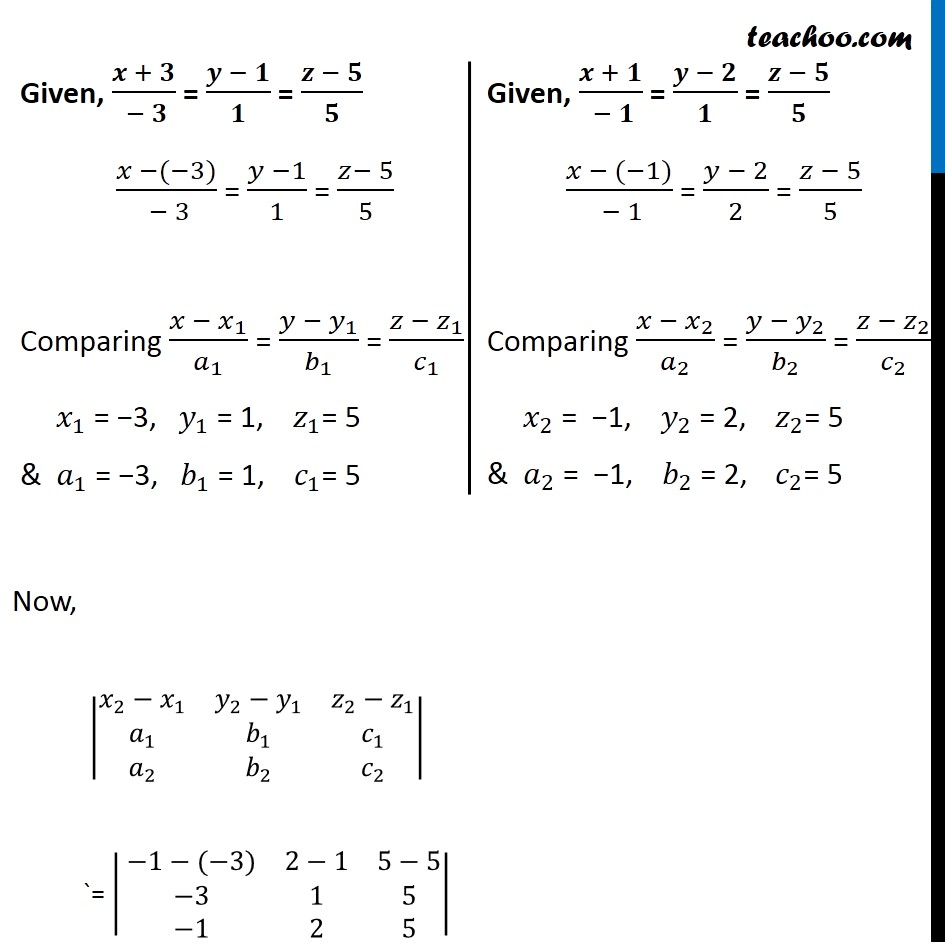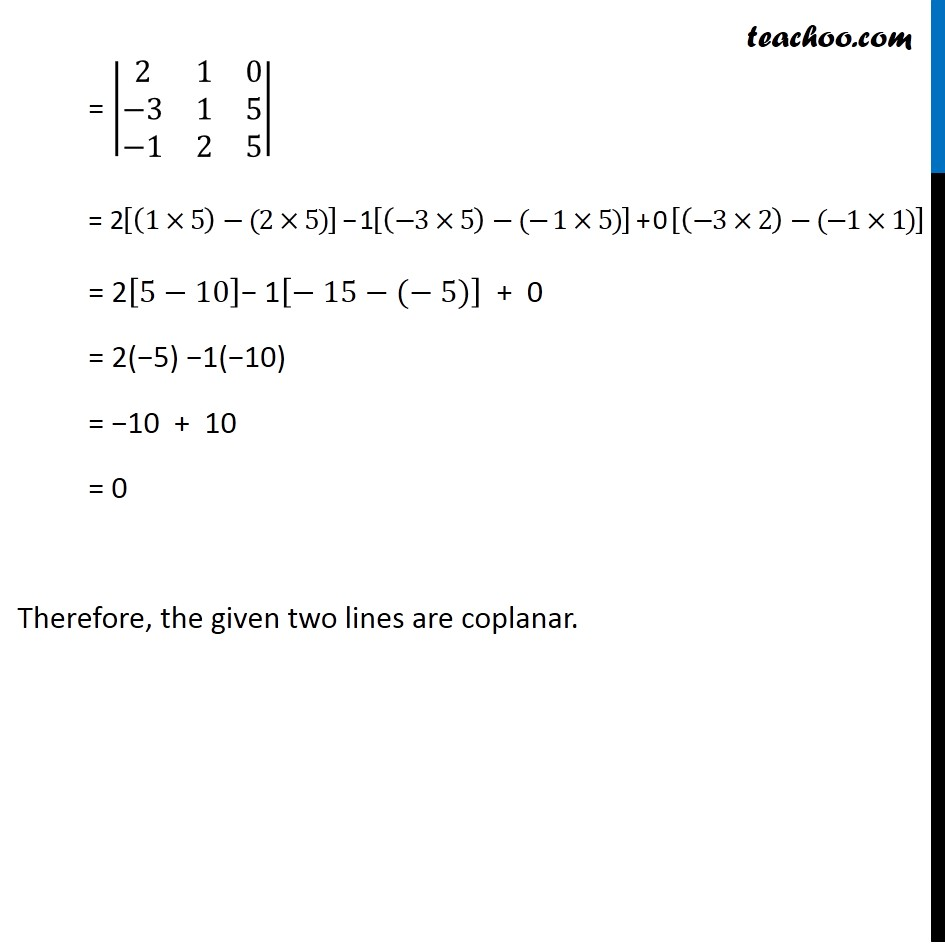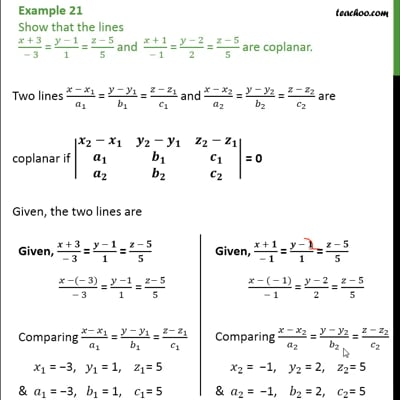Examples

Chapter 11 Class 12 Three Dimensional Geometry
Serial order wiseThis video is only available for Teachoo black users

Solve all your doubts with Teachoo Black (new monthly pack available now!)

### Transcript

Example 21 Show that the lines (𝑥 + 3)/( −3) = (𝑦 − 1)/1 = (𝑧 − 5)/5 and (𝑥 + 1)/( −1) = (𝑦 − 2)/2 = (𝑧 − 5)/5 are coplanar. Two lines (𝑥 − 𝑥_1)/𝑎_1 = (𝑦 − 𝑦_1)/𝑏_1 = (𝑧 − 𝑧_1)/𝑐_1 and (𝑥 − 𝑥_2)/𝑎_2 = (𝑦 − 𝑦_2)/𝑏_2 = (𝑧 − 𝑧_2)/𝑐_2 are coplanar if |■8(𝒙_𝟐−𝒙_𝟏&𝒚_𝟐−𝒚_𝟏&𝒛_𝟐−𝒛_𝟏@𝒂_𝟏&𝒃_𝟏&𝒄_𝟏@𝒂_𝟐&𝒃_𝟐&𝒄_𝟐 )| = 0 Given, the two lines are Given, (𝒙 + 𝟑)/( − 𝟑) = (𝒚 − 𝟏)/𝟏 = (𝒛 − 𝟓)/𝟓 (𝑥 −(−3))/( − 3) = (𝑦 −1)/1 = (𝑧− 5)/5 Comparing (𝑥 − 𝑥_1)/𝑎_1 = (𝑦 − 𝑦_1)/𝑏_1 = (𝑧 − 𝑧_1)/𝑐_1 𝑥_1 = −3, 𝑦_1 = 1, 𝑧_1= 5 & 𝑎_1 = −3, 𝑏_1 = 1, 𝑐_1= 5 Given, (𝒙 + 𝟏)/( − 𝟏) = (𝒚 − 𝟐)/𝟏 = (𝒛 − 𝟓)/𝟓 (𝑥 − (−1))/( − 1) = (𝑦 − 2)/2 = (𝑧 − 5)/5 Comparing (𝑥 − 𝑥_2)/𝑎_2 = (𝑦 − 𝑦_2)/𝑏_2 = (𝑧 − 𝑧_2)/𝑐_2 𝑥_2 = −1, 𝑦_2 = 2, 𝑧_2= 5 & 𝑎_2 = −1, 𝑏_2 = 2, 𝑐_2= 5 Now, |■8(𝑥_2−𝑥_1&𝑦_2−𝑦_1&𝑧_2−𝑧_1@𝑎_1&𝑏_1&𝑐_1@𝑎_2&𝑏_2&𝑐_2 )| `= |■8( −1−(−3)&2−1&5−5@ −3&1&5@ −1&2&5)| = |■8(2&1&0@−3&1&5@−1&2&5)| = 2[(1×5)−(2×5)] − 1[(−3×5)−(− 1×5)] + 0 [(−3×2)−(−1×1)] = 2[5−10]− 1[− 15−(− 5)] + 0 = 2(−5) −1(−10) = −10 + 10 = 0 Therefore, the given two lines are coplanar.